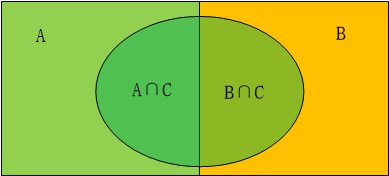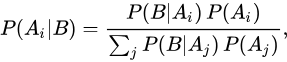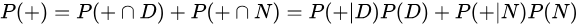# 最大似然估计

## 求模型参数

例子

## 应用

例子1

# 最大后验估计

## 贝叶斯公式

$P(Girl|Pants)=\frac{P(Girl)*P(Pants|Girl)}{P(Boy)*P(Pants|Boy)+P(Girl)*P(Pants|Girl)}$ 式1

$P(B|A)=\frac{P(B)*P(A|B)}{P(B')*P(A|B')+P(B)*P(A|B)}$ —-（B’为B的互补，例如男生女生） 式2

$P(B|A)=\frac{P(A,B)}{P(A)}$ 式3 又可以写为

$P(B|A)*P(A)=P(A,B)$ 式4

P(A|B)*P(B) = P(B|A)*P(A)，即：

$P(A|B)=\frac{P(A)*P(B|A)}{P(B)}$ 式5## 贝叶斯推断

### 什么是贝叶斯推断

• P(D)代表雇员吸毒的概率，不考虑其他情况，该值为0.005。因为公司的预先统计表明该公司的雇员中有0.5%的人吸食毒品，所以这个值就是D的先验概率。
• P(N)代表雇员不吸毒的概率，显然，该值为0.995，也就是1-P(D)。
• P(+|D)代表吸毒者阳性检出率，这是一个条件概率，由于阳性检测准确性是99%，因此该值为0.99。
• P(+|N)代表不吸毒者阳性检出率，也就是出错检测的概率，该值为0.01，因为对于不吸毒者，其检测为阴性的概率为99%，因此，其被误检测成阳性的概率为1 - 0.99 = 0.01。
• P(+)代表不考虑其他因素的影响的阳性检出率。该值为0.0149或者1.49%。我们可以通过全概率公式计算得到：此概率 = 吸毒者阳性检出率（0.5% x 99% = 0.495%)+ 不吸毒者阳性检出率（99.5% x 1% = 0.995%)。P(+）=0.0149是检测呈阳性的先验概率。用数学公式描述为：$P(D|-)=\frac{P(-|D)P(D)}{P(-|D)P(D)+P(-|N)P(N)}$

=$\frac{0.01×0.005}{0.01×0.005+0.99×0.995}$

≈0.0000507

### 贝叶斯推断与拼接纠正

P(S|t)名义上是指我们猜测的单词t是用户真正想输入单词的概率，不同的单词概率不同，这就涉及到最大似然估计。例如用户输入的单词是thriw,这时throw跟thraw都有可能，但是你会想到，o跟i很接近，用户可能要输的单词是throw的可能性比thraw的可能性大得多，根据最大似然估计找出最可能的单词。但是，有时候光有最大似然并不能完美的解决问题，我们还需要利用先验概率P(t)

P(t)使我们猜测的单词出现的概率，这些单词t1、t2、t3….理论上有无穷种，但它是一种先验概率，对于单词来说，可能有点抽象。这里举一个分词的例子：

The girl saw the boy with a telescope.